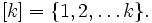# Boolean-valued function

A boolean-valued function is a function of the typewhereis an arbitrary set and where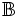is a boolean domain.
A binary sequence is a boolean-valued function, where. In other words,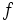is an infinite sequence of 0's and 1's.
A binary sequence of length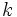is a boolean-valued function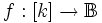, where